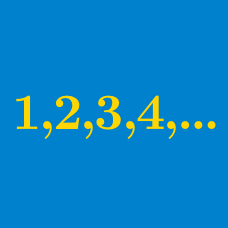Algebra

# Arithmetic Progressions - Problem Solving

Non-zero numbers $a, b, c, d, e$ form an arithmetic progression. If $\frac{b+d}{2} + \frac{a+e}{4} = kc,$ find the value of $k$.

Two sequences $\{ x, a_1, a_2, a_3, y \}$ and $\{ x, b_1, b_2, b_3, b_4, b_5, y \}$ each form an arithmetic progression. If $x \neq y$, what is the value of

$\frac{a_2 - a_1}{b_5 - b_4} ?$

For an arithmetic progression $\{a_n\}$, the following equalities hold: $a_3 + a_5 = 36, \quad a_2 a_4 = 180.$

Find the largest $n$ such that $a_n < 100.$

An arithmetic progression $\{a_n \}$ satisfies $a_2 + a_4 + a_6 + \cdots + a_{2n} = 2n^2 + 3n.$ Find the value of $a_3 + a_6 + a_9 + \cdots + a_{18}.$

For an arithmetic progression $\{ a_n \}$, $a_3 = 8$ and $a_{10} = 29$. If $a_n = 200$, what is $n ?$

×